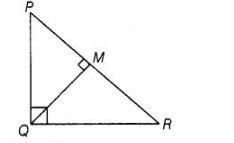# In a ΔPQR, PR2 – PQ2 = QR2 and M is a point

Question:

In a ΔPQR, PR2 – PQ2 = QR2 and M is a point on side PR such that QM ⊥ PR.

Prove that QM2 =PM × MR.

Solution:

Given In A PQR,                             PR2 – PQ2 = QR2 and QM ⊥ PR

To prove                                         QM2 = PM x MR

Proof Since,                        PR2 – PQ2 = QR2

⇒                                                     PR2 = PQ2 + QR2So, $\triangle P Q R$ is right angled triangle at $Q$.

In $\Delta Q M R$ and $\Delta P M Q$,  $\angle M=\angle M$ [each 90°]

$\angle M Q R=\angle Q P M \quad$ [each equal to $90^{\circ}-\angle R$ ]

$\therefore$ $\Delta Q M R \sim \Delta P M Q$ [by AAA similarity criterion]

Now, using property of area of similar triangles, we get

$\frac{\operatorname{ar}(\Delta Q M R)}{\operatorname{ar}(\Delta P M Q)}=\frac{(Q M)^{2}}{(P M)^{2}}$

$\Rightarrow \quad \frac{\frac{1}{2} \times R M \times Q M}{\frac{1}{2} \times P M \times Q M}=\frac{(Q M)^{2}}{(P M)^{2}} \quad\left[\because\right.$ area of triangle $=\frac{1}{2} \times$ base $\times$ height $]$

$\Rightarrow$ $Q M^{2}=P M \times R M$

Hence proved.